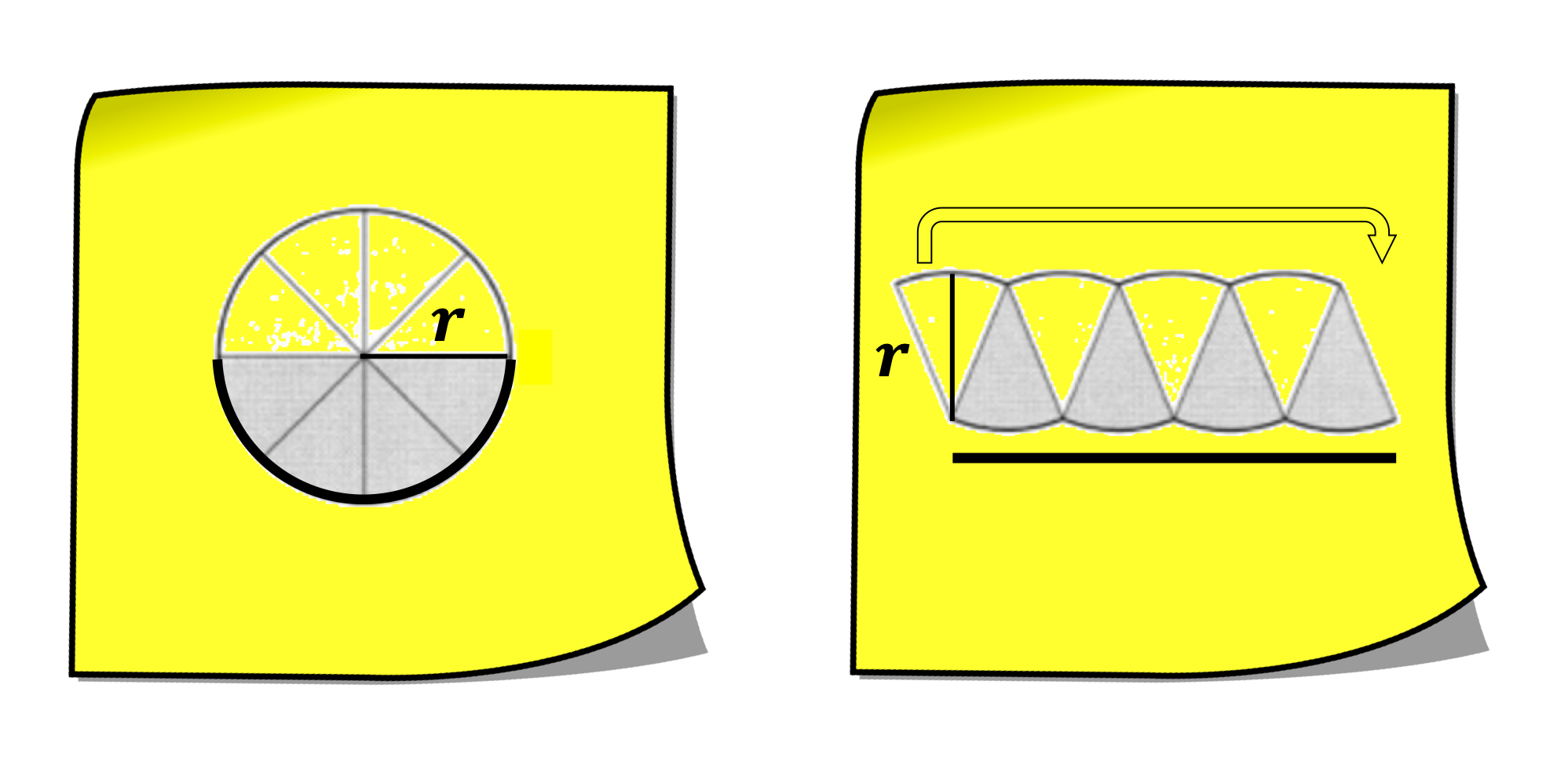Select Page
Area of a Circle Related to the Area of a Rectangle / Parallelogram

This Sticky Math Lesson examines the area of a circle conceptually by connecting to the area of rectangle / parallelogram. It is helpful to examine the relationship between the area of a rectangle and any parallelogram before this lesson. Incidentally, this lesson is a good example of stopping to contextualize (MP2) in each of the permutations of the area equation. The lesson can introduce vocabulary through the drawings, offers an opportunity for students to use precise vocabulary (MP6), and acts as a formative assessment of academic vocabulary.  7.G.B.4

Start Making Connections Now!

Any connection students make throughout the lesson should be valued and acknowledged to build students’ mathematical agency, authority and identity; however, the idea for this stage in the lesson is to realize:

Both stickies represent the the area of a circle

Shading is not representative of the entire area of the circle; it represents half the area of the circle.

The right sticky has the area of circle organized so it is similar to a parallelogram, or a rectangle if you think of moving half of an eighth (1 sixteenth).  [Alternately, you can think of cutting the circle into infinitely smaller and smaller pieces; here is a sample.]

On the right sticky, the height is equivalent to the radius of the circle on the left sticky.

On the right sticky, the length is equivalent to half of the circumference.A third sticky is used to make connections to the abstract, symbolic representation of the relationship found in the previous Sticky Math Pair:

All three stickies represent the the area of a circle

The middle sticky has the area of circle organized so it is similar to a parallelogram.

On the middle sticky, the height is equivalent to the radius of the circle on the right sticky.

On the middle sticky, the length is equivalent to half of the circumference of the circle on the right sticky.It would be helpful to display the stickies in this step with the stickies from the previous step; better yet, keeping a visual record of the entire process would be ideal.

All three stickies represent the the area of a circle

The circumference is equivalent to 2(pi)r or stated more conceptually 2r, pi times.

The circumference has been replaced with 2(pi)rWe are almost there, but will students understand WHY the area of a circle is pi times r squared?

On the right sticky, moving a sixteenth, half of an eighth, creates a rectangle.

On the right sticky, the radius is the height of the rectangle.

On the right sticky, the length is pi times r or stated more conceptually r pi times.We have arrived at the customary symbolic notation of the area of a circle; however, what does it mean?  How does it relate to area that is usually noted in square units?

The superimposed rectangles cover the same area as the circle.

As we have previously, rather than reading the expression on the right side of the equation as pi times r squared, think of it as r squared pi times (think of pi as the coefficient of the square term). [Left sticky]

In other words, pi number of squares with the radius as a side length create a rectangle that covers the same area as the circle.  [Right sticky]Start Making Connections Now!